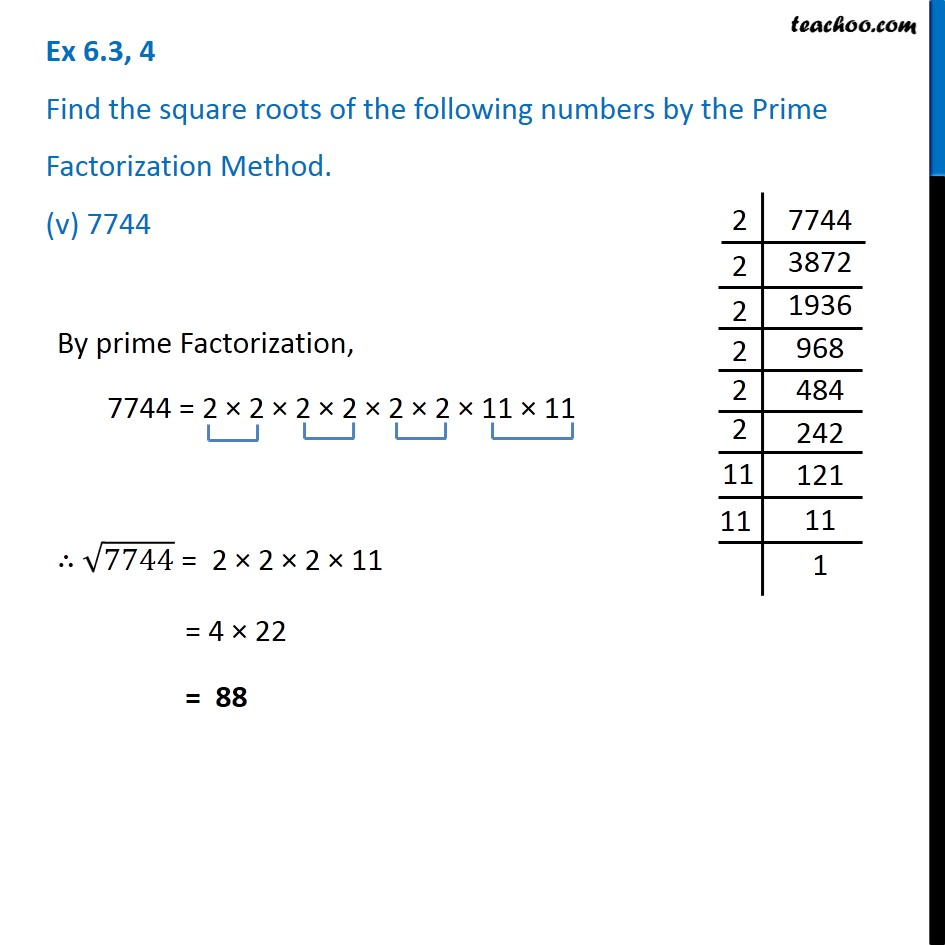Ex 6.3

Chapter 6 Class 8 Squares and Square Roots
Serial order wisex

### Transcript

Ex 6.3, 4 Find the square roots of the following numbers by the Prime Factorization Method. (v) 7744 By prime Factorization, 7744 = 2 × 2 × 2 × 2 × 2 × 2 × 11 × 11 ∴ √7744 = 2 × 2 × 2 × 11 = 4 × 22 = 88